# 5.2 Types of Operators

#### 5.2.1 Arithmetic Operators

Arithmetic operators take numerical values (either literals or variables ) as their operands and return a single numerical value. The standard arithmetic operators are addition (+), subtraction ( “), multiplication (*), and division (/). See Table 5.2.

##### Table 5.2. Arithmetic operators.

Operator/Operands

Function

x + y

x “ y

Subtraction

x * y

Multiplication

x / y

Division [a]

x % y

Modulus

[a] The / operator returns a floating-point division in JavaScript, not a truncated division as it does in languages such as C or Java. For example, 1/2 returns 0.5 in JavaScript and 1/2 returns 0 in Java.

##### Example 5.3
` <html>     <head><title>Arithmetic Operators</title></head>     <body>     <h2>Arithmetic operators</h2>     <p> 1   <script language="JavaScript"> 2       var num1 = 5;         var num2 = 7; 3  var result = num1 + num2;  4       document.write("<h3>num1 + num2 = "+  result  ); 5  result = result + (10 / 2 + 5);  6       document.write("<h3>12 + (10 / 2 + 5) = " +  result  ); 7   </script>     </body>     </html> `

EXPLANATION

1. This is the start of a JavaScript program.

2. Variables num1 and num2 are declared and assigned values 5 and 7, respectively.

3. The variable result is assigned the sum of num1 and num2 .

4. The results are displayed by the browser. Note that the + sign in this expression is used to concatenate two strings. When a string is concatenated to a number, JavaScript converts the number to a string. The value stored in the variable result is converted to a string and joined to the string on the left-hand side of the + sign.

5. The expression on the right-hand side of the = sign is evaluated and assigned to the variable, result, on the left-hand side of the = sign. (The parentheses are not needed, but used for clarity.) The browser output is shown in Figure 5.3.

##### Figure 5.3. Ouput from Example 5.3.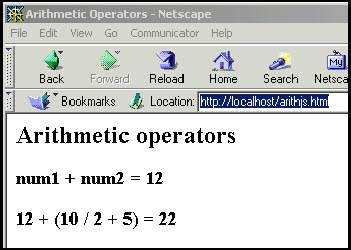#### 5.2.2 Shortcut Assignment Operators

The shortcut assignment operators allow you to perform an arithmetic or string operation by combining an assignment operator with an arithmetic or string operator. For example, x = x + 1 can be written x+=1 . See Table 5.3.

##### Table 5.3. Assignment operators.

Operator

Example

Meaning

=

var x = 5;

Assign 5 to variable x.

+=

x += 3;

Add 3 to x and assign result to x.

“=

x “= 2;

Subtract 2 from x and assign result to x.

*=

x *= 4;

Multiply x by 4 and assign result to x.

/=

x /= 2;

Divide x by 2 and assign result to x.

**=

x **= 2;

Square x and assign result to x .

%=

x %= 2

Divide x by 2 and assign remainder to x.

##### Example 5.4
` <html>     <head>     <title>Assignment and Shortcut Operators</title> 1   <script language = "JavaScript"> 2  var num=10;  3       document.write("<font size='+1'>" + "<br>" +                "num is assigned " + 10); 4  num += 2;  document.write("<br>num += 2; num is " + num ); 5  num -= 1;  document.write("<br>num -= 1; num is " + num); 6  num *= 3;  document.write("<br>num *= 3; num is " + num); 7  num %= 5;  document.write("<br>num %= 5; num is " + num); 8   </script>     </head>     <body bgcolor="yellow" text="blue"> `

EXPLANATION

1. JavaScript program starts here.

2. The variable num is assigned 10 .

3. Output is sent to the browser.

4. The shortcut assignment operator, +=, adds 2 to the variable num. This is equivalent to num = num + 1; .

5. The shortcut assignment operator, “=, subtracts 1 from the variable num. This is equivalent to num = num - 1; .

6. The shortcut assignment operator, *, multiplies the variable num by 3. This is equivalent to num = num * 3; .

7. The shortcut assignment modulus operator, %, yields the integer amount that remains after the scalar num is divided by 5. The operator is called the modulus operator or remainder operator. The expression var%=5 is equivalent to num = num % 5 ;.

8. JavaScript ends here. The output is shown in Figure 5.4.

##### Figure 5.4. Output from Example 5.4.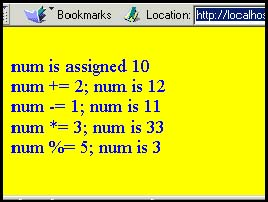#### 5.2.3 Autoincrement and Autodecrement Operators

To make programs easier to read, to simplify typing, and, at the machine level, to produce more efficient code, the autoincrement (++) and autodecrement ( “ “) operators are provided.

The autoincrement operator performs the simple task of incrementing the value of its operand by 1, and the autodecrement operator decrements the value of its operand by 1. The operator has two forms: the first form prefixes the variable with either ++ or “ “ (e.g., ++x or “ “ x) ; the second form postfixes (places the operator after) the variable name with either ++ or “ “ (e.g., x++ or x “ “). For simple operations, such as x++ or x “ “, ++x or “ “x, the effect is the same; both ++x and x++ add one to the value of x, and both “ “ x and x “ “ subtract one from the value of x .

Now you have four ways to add 1 to the value of a variable:

• x = x + 1;

• x += ;

• x++;

• ++x;

and four ways to subtract 1 from the value of a variable:

• x = x “ 1;

• x “= 1;

• x “ “;

• “ “x;

Refer to Table 5.4. In "Loops" on page 101, you'll see these operators are commonly used to increment or decrement loop counters.

##### Table 5.4. Autoincrement and autodecrement operators.

Operator

Function

What It Does

Example

++x

Pre-increment

x = 3; x++;

x is now 4

x++

Post-increment

x = 3; ++x;

x is now 4

“ “x

Pre-decrement

Subtracts 1 from x

x = 3; x “ “ ;

x is now 2

x “ “

Post-decrement

Subtracts 1 from x

x = 3; “ “x;

x is now 2

##### Autoincrement and Autodecrement Operators and Assignment

The placement of the operators does make a difference in more complex expressions, especially when part of an assignment; for example, y = x++ is not the same as y = ++x .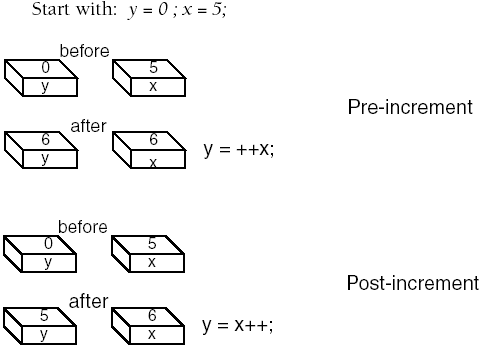##### Example 5.5
` <html>     <head><title>Auto-increment and Auto-decrement</title></head>     <body>         <script language = "JavaScript"> 1  var x=5;   var y=0;  2  y = ++x;  //  add one to x first; then assign to y  document.write("<h3>Pre-increment:<br>"); 3           document.write("y is " +  y + "<br>");             document.write("x is " + x + "<br>");             document.write("-----------------------<br>"); 4  var x=5;   var y=0;  5  y=x++;  //  assign value in x to y; then add one to x  document.write("<h3>Post-increment:<br>"); 6           document.write("y is " +  y  + "<br>");             document.write("x is " +  x  + "<br></h3>");         </script>     </body>     </html> `

EXPLANATION

1. The variables, x and y , are intialized to 5 and 0, respectively.

2. The pre-increment operator is applied to x . This means that x will be incremented before the assignment is made. The value of x was 5 , now it is 6 . The variable y is assigned 6. x is 6, y is 6 .

3. The new values of y and x are displayed in the browser window.

4. The variables x and y are assigned values of 5 and 0, respectively.

5. This time the post-increment operator is applied to x . This means that x will be incremented after the assignment is made. The number 5 is assigned to the variable y , and then x is incremented by 1 . So x is 5 and y is 6 .

6. The new values of y and x are displayed in the browser window. See Figure 5.5.

##### Figure 5.5. Output from Example 5.5.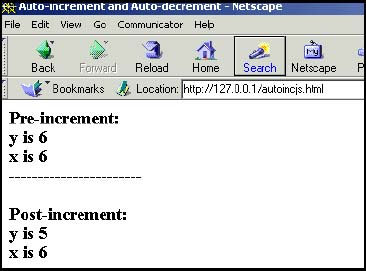#### 5.2.4 Concatenation Operator

As shown in previous examples, the + sign is used for concatenation and addition. The concatenation operator, the + sign, is a string operator used to join together one or more strings. In fact, the concatenation operator is the only operator JavaScript provides to manipulate strings.

In the example, "correct" + "tion" + "al" , the result is "correctional" . If the operands are a mix of strings and numbers , JavaScript will convert the numbers to strings . For example, "22" + 8 results in "228" , not 30 . If the operands are numbers, then the + sign is the addtion operator as in 5 + 4 . But suppose we say, "22" * 1 + 4 . In this case, JavaScript sees the multiplication operator (*) and converts the string "22" to a number, resulting in 22 + 4 or 26 . Netscape Navigator provides the JavaScript console for testing these expressions or you can type javascript: in the URL, followed by the expression you want to test, as shown in Figures 5.6 and 5.7.

##### Figure 5.6. Evaluating expressions in the javascript: URL. The result of the test, 26 , is displayed in the browser window.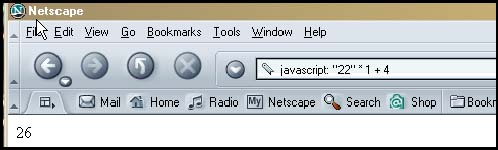##### Figure 5.7. Concatenation of a string and a number.The concatenation operator is summarized in Table 5.5. To explicitly convert strings to numbers, JavaScript provides built-in functions called parseInt() and parseFloat() , discussed in Sections 5.3.1 and 5.3.2, respectively.

##### Table 5.5. The concatenation operator.

Operator

Example

Meaning

+

"hot" + "dog"

Concatenates (joins) two strings; creates "hotdog" .

"22" + 8

Converts number 8 to string "8" , then concatenates resulting in "228" . In statements involving other operators, JavaScript does not convert numeric values to strings.

+=

x ="cow"; x += "boy";

Concatenates two strings and assigns the result to x; x becomes "cowboy" .

#### 5.2.5 Comparison Operators

When operands are compared, relational and equality operators are used. The operands can be numbers or strings. The result of the comparison is either true or false ”a Boolean value. Strings are compared letter by letter (lexographically) using Unicode  values to represent the numeric value of each letter; thus, "A" is less than "B" , and when comparing "Daniel" with "Dan", "Daniel" is greater than "Dan" . When comparing strings, JavaScript pads "Dan" with three spaces to make it the same length as " Daniel" . Refer to Table 5.6.

 Unicode is not supported in versions of JavaScript prior to 1.3. Unicode is compatible with ASCII characters. The first 128 Unicode characters correspond to the ASCII character set and have the same byte value.

##### Table 5.6. Comparison operators.

Operator/Operands

Function

x == y

x is equal to y

x != y

x is not equal to y

x > y

x is greater than y

x >= y

x is greater than or equal to y

x < y

x is less than y

x <= y

x is less than or equal to y

x = = = y

x is identical to y in value and type

x != = y

x is not identical to y

##### What Is Equal?

In an ideal world, there would be equality between the sexes and among the races and religions, but in the real world equality is a debatable topic, often determined by governments . In JavaScript, operators determine the equality or inequality of their operands, based on more specific rules. When using the == or != equality operators, the operands may be of any given data type ”numbers, strings, Booleans, objects, arrays, or a combination of these ”and there are rules that govern whether they are equal. For example, two strings are equal when they have the same sequence of characters, same length, and same characters in corresponding positions . Two numbers are equal when they have the same numeric value. If a string is compared with a number, they are equal if the number has the same characters as the string; for example, "500" is equal to 500. NaN (Not a Number) is not equal to anything, including NaN . Positive and negative zeros are equal. Two objects are equal if they refer to the same object. Two Boolean operands are equal if they are both true or both false. Null and undefined types are equal. To test any of the expressions shown in Table 5.7, use the JavaScript Console. Figure 5.8 shows an example using Netscape.

##### Figure 5.8. Testing the equality of two strings. Is "William" equal to "william" ? Nope.##### Table 5.7. Equality test with strings and numbers.

Test

Are They Equal?

"William" == "William"

true

"william" == "William"

false

5 == 5.0

true

"54" == 54

true

"5.4" == 5.4

true

NaN == NaN

false

null == null

true

“0 == +0

true

false == false

true

true == 1

true

null == undefined

true

##### What Is Identical?

Men are equal; clones are identical. The === and !== equality operators test that its operands are not only of the same value, but also of the same data type. String "54" is equal to number 54 , but not identical because one is a string and the other is a number, even though their values are equal. See Table 5.8.

##### Table 5.8. Identity test with strings and numbers.

Test

Are They Equal?

"William" === "William"

true

"william" == "William"

false

5 == 5.0

true

"54" === 54

false

NaN == NaN

false

null == null

true

-0 == +0

true

false == false

true

true == 1

false

null == undefined

false

##### Comparing Numbers

When the comparison operators are used to compare numbers, numeric values are compared; as in, is 50 > 45 ? A Boolean value of either true or false is returned.

 x > y x is greater than y x >= y x is greater than or equal to y x < y x is less than y x <= y x is less than or equal to y
##### Example 5.6
` <html>     <head>     <title>Comparing Numbers</title>     </head><body> 1       <script language = "JavaScript"> 2           var x = 5;             var y = 4; 3  var result = x > y;  4           document.writeln("<h3>The result is "+  result  + ".<br>"); 5  result = x < y;  6           document.writeln( "The result is " +  result  + ".<br>"); 7       </script>     </body>     </html> `

EXPLANATION

1. The JavaScript program starts here.

2. The variables x and y are assigned values to be compared later in the program.

3. If the value of x is greater than the value of y , a Boolean value of either true or false is returned and assigned to the variable result .

4. The Boolean result of the comparison is displayed by the browser. It is true; x is greater than y .

5. If x is less than y, true is assigned to the variable, result ; otherwise it is assigned false .

6. The Boolean result of the comparison is displayed by the browser. It is false; x is not greater than y .

7. This tag marks the end of the JavaScript program. The output is shown in Figure 5.9.

##### Figure 5.9. Output from Example 5.6.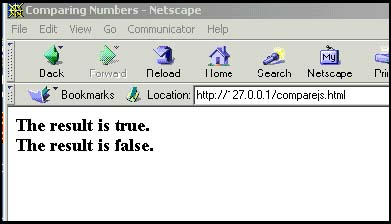##### Comparing Strings

The difference between comparing strings and numbers is that numbers are compared numerically and strings are compared alphabetically , based on the ASCII character set. The strings are compared letter by letter, from left to right, and if they are exactly the same all the way to end, they are equal. Once a letter in one string differs from the corresponding letter in the second string, the comparison stops and each of the differing letters is evaluated. For example, if the string "Dan" is compared with "dan" , the comparison stops at the first letters D and d. "Dan" is smaller than "dan" , because the letter D has a lower ASCII value than the letter d. D has an ASCII decimal value of 68, and d has an ASCII value of 100.

To avoid the case-sensitivity issue when comparing strings, JavaScript provides the built-in string functions, toUpperCase() and toLowerCase(), discussed in "Free Form and Reserved Words" on page 17 and Table 9.10 on page 184.

 "string1" > "string2" "string1" is greater than "string2" "string1" >= "string2" "string1" is greater than or equal to "string2" "string1" < "string2" " string1" is less than "string2" "string1" <= "string2" "string1" is less than or equal to "string2"
##### Example 5.7
` <html>     <head>     <title>Comparing Strings</title>     </head><body> 1       <script language = "JavaScript"> 2           var fruit1 = "pear";             var fruit2 = "peaR"; 3  var result = fruit1 > fruit2;  4           document.writeln( "<h3>The result is "+  result  + ".<br>"); 5  result = fruit1 < fruit2;  6           document.writeln( "The result is " + result + ".<br>"); 7  result = fruit1 === fruit2;  //  Are they identical; i.e., value and type are the same?  8           document.writeln( "The result is " + result + ".<br>");         </script>     </body>     </html> `

EXPLANATION

1. This is the start of the JavaScript program.

2. The variables, fruit1 and fruit2 , are assigned to string values, differing by only one letter.

3. The string values are compared and a Boolean value of true or false will be returned and assigned to the variable, result. "pear" is greater than "peaR" because the r has an ASCII value of 114 and the R has an ASCII value of 82.

4. The result of the comparison in line 3 is true and the result is sent to the browser.

5. This time "pear" is compared to "peaR" with the less than operator. The result is false .

6. The result of the comparison in line 5 is false and the result is sent to the browser.

7. The identical equality operator is used. Since the strings are not identical, the result is false.

8. The result of the comparison in line 7 is false and the result is sent to the browser. The output of the script is shown in Figure 5.10.

##### Figure 5.10. Output from Example 5.7.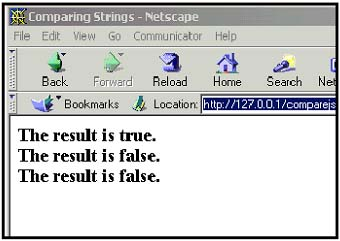#### 5.2.6 Logical Operators

The logical operators allow you combine the relational operators into more powerful expressions for testing conditions and are most often used in if statements. They evaluate their operands from left to right, testing the Boolean value of each operand in turn : Does the operand evaluate to true or false? In the expression if ( x > 5 && x < 10 ), the && is a logical operator. The expression simplified means, "if x is greater than 5 and x is also less than 10, then do something"; in the case of the logical AND ( && ), if the first expression returns true and the second expression also returns true , then the whole expression is true. If instead of && we used , the operator means OR and only one of the expressions must be true.

Sometimes the result of a test is not Boolean. When logical operators have numeric operands, such as 5 && 6 , the result of the entire expression is the value of the last evaluated expression. A numeric operand is true if it evaluates to any number that is not zero. 5, “2, and 74 are all true. is false. For example, when using the && (AND) operator, both operands must be true for the whole expression to be true. The value returned from an expression such as 5 && 6 is 6 , the last value evaluated by the operator. 5 is not zero (true) and 6 is not zero (true), therefore, the expression is true. 5 && 0, 0 && 0 and 0 && 5 all yield , which is false. See Table 5.9.

The three logical operators are the logical AND, logical OR, and logical NOT. The symbol for AND is && , the symbol for OR is , and the symbol for NOT is ! .

##### Table 5.9. Logical operators and their functions.

Operator/Operands

Function

num1 && num2

True, if num1 and num2 are both true. Returns num1 if evaluated to false; otherwise returns num2 . If operands are Boolean values, returns true if both operands are true; otherwise returns false.

num1 num2

True, if num1 is true or if num2 is true.

! num1

Not num1; true if num1 is false; false if num1 is true.

##### The && Operator (Logical AND)

We all know the meaning of the English statement, "If you have the money and I have the time...." Whatever is supposed to happen is based on two conditions, and both conditions must be met. You must have the money and I must have the time. JavaScript uses the symbol && to represent the word AND. This operator is called the logical AND operator. If the expression on the left-hand side of the && evaluates to zero, null, or the empty string "", the expression is false. If the expression on the left-hand side of the operator evaluates to true ( non-zero ), then the right-hand side is evaluated, and if that expression is also true, then the whole expression is true. If the left-hand side evaluates to true, and the right-hand side is false, the expression is false. If evaluated as Booleans, the same rules apply, except the returned value will be either Boolean true or false . See Table 5.10.

##### Table 5.10. Logical AND examples.

Expression

What It Evaluates To

true && false

false

true && true

true

"honest" && true

true

true && ""

(empty string)

true && "honest"

honest

5 && 0

5 && “6

“6

5 && false

false

null && 0

null

null && ""

null

null && false

null

"hello" && true && 50

50

"this" && "that"

that

##### Example 5.8
` <html>     <head><title>Logical AND Operator</title>     </head>     <body bgcolor="lightblue">     <font="+1">     <script language="JavaScript"> 1       var answer = prompt("How old are you? ", ""); 2  if ( answer > 12 && answer < 20 )  {            alert("Teenagers rock!");         }     </script>     </body>     </html> `

EXPLANATION

1. The user is prompted for his age. The variable called answer is assigned the value he enters. (See Figure 5.11.)

##### Figure 5.11. The user enters his age.2. If the value of answer is greater than 12 and also less than 20, the statement enclosed within the curly braces is executed: an alert box appears displaying Teenagers rock! (See Figure 5.12.) If the user enters any other value, nothing happens.

##### Figure 5.12. If the user enters his age and it is greater than 12 and less than 20, this alert box appears.##### The Operator (Logical OR)

In the English statement "If you have some cash or I have a credit card..." the word or is used in the condition. With the or, only one of the conditions must be met (hopefully that you have the cash!). JavaScript uses the symbol to represent the logical OR. If the expression on the left-hand side of the operator is evaluated as true (non-zero), the value of the expression is true, and no further checking is done. If the value on the left-hand side of the operator is false, the value of the expression on the right-hand side of the operator is evaluated, and if true, the expression is true; that is, only one expression must be true. Once an expression returns true, the remaining expressions can be either true or false. It doesn't matter, as long as one expression is true . Refer to Table 5.11.

##### Table 5.11. Logical OR examples.

Expression

What It Evaluates To

true false

true

true true

true

"honest" true

honest

true && ""

true

true "honest"

true

5 0

5

5 “6

5

5 false

5

null 0

null ""

(empty string)

null false

false

"hello" true 50

hello

"this" "that"

this

##### Example 5.9
` <html>     <head>     <title>Logical OR Operator</title>     </head>     <body bgcolor="lightblue">     <font="+1">     <script language="JavaScript"> 1       var answer = prompt("Where should we eat? ", ""); 2       if ( answer == "McDonald's"  answer == "Taco Bell"               answer == "Wendy's"){ 3               alert("No fast food today, thanks.");            }     </script>     </body>     </html> `

EXPLANATION

1. The user is prompted to choose a place to eat. The variable called answer is assigned the value he enters. (See Figure 5.13.)

##### Figure 5.13. The user enters a value.2. If the value of answer is any one of McDonald's or Taco Bell or Wendy's , the statement enclosed within the curly braces, is executed: an alert box appears displaying No fast food today, thanks . (See Figure 5.14.) If he enters any other value, nothing happens.

##### Figure 5.14. If the user enters any one of the values in line 2, this alert box appears.##### The ! Operator (Logical NOT)

In the English statement "That's not true!" the word not is used for negation: not true is false, and not false is true. JavaScript provides the NOT ( ! ) operator for negation. The ! operator is called a unary operator because it has only one operand; for example, ! true or ! 5 . It returns true if the expression evaluates to false and returns false if the expression evaluates to true. See Table 5.12.

##### Table 5.12. Logical NOT examples.

Expression

What It Evaluates To

! "this"

false

! 0

true

!2

false

! false

true

! null

true

! undefined

true

##### Example 5.10
` <html>     <head>     <title>Logical NOT Operator</title>     </head>     <body bgcolor="lightblue">     <font="+1">     <script language="JavaScript"> 1       var answer = true; 2       alert("Was true. Now " +  ! true  );     </script>     </body>     </html> `

EXPLANATION

1. The Boolean value true is assigned to the variable answer .

2. The expression sent to the alert dialog box, ! true , negates the value true , (not true) making it false . (See Figure 5.15.)

##### Figure 5.15. The ! operator caused true to become false.In summary, the following example illustrates the logical operators and the values they return.

##### Example 5.11
` <html>     <head>     <title>Logical (Boolean) Operators</title>     </head>     <body>     <script language = "JavaScript"> 1       var num1=50;         var num2=100;         var num3=0; 2       document.write("<h3>num1 && num2 is " + (  num1 && num2  ) +             ".<br>"); 3       document.write("num1  \$num2 is " + (  num1  num2  ) +".<br>"); 4       document.write("! num1 is " +  !num1  +".<br>"); 5       document.write("!(num1 && num2) is " +  !(num1 && num2)  +             ".<br>"); 6       document.write("!(num1 && num3) is " +  !(num1 && num3)  +             ".<br>");     </script>     </body>     </html> `

EXPLANATION

1. Three variables, num1, num2 , and num3, are initialized .

2. The && operator expects both of its operands to be true, if the expression is to be true. A true value is any number that is not zero. In the expression, 50 && 100 , both operands are true. The value of the last true operand, 100 , is returned.

3. The operator expects only one of its operands to be true if the whole expression is to be true. 50 100 is true because the first operand evaluates to a non-zero value. Because 50 is true and only one operand must be true, the evaluation stops here and 50 is returned.

4. The ! (NOT) operator negates its operand. ! 50 means ! true ; that is, false.

5. Because the expression num1 && num2 is enclosed in parentheses, it is evaluated first, resulting in 50 && 100, true . Then the ! (NOT) operator evaluates ! (true), resulting in Boolean false .

6. The expression, num1 && num3 , enclosed in parentheses, is evaluated first. Since num3 is , the expression evaluates to false. ! (false) is true . See Figure 5.16.

##### Figure 5.16. Output from Example 5.11.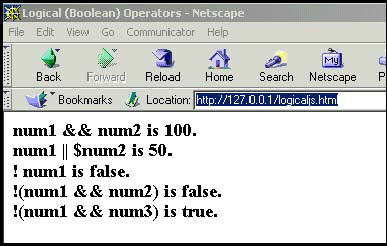#### 5.2.7 The Conditional Operator

The conditional operator is called a ternary operator because it requires three operands. It is often used as a shorthand method for if/else conditional statements. (See Chapter 6, "Under Certain Conditions.") Although we cover if/else in Chapter 6, the format below shows both the conditional operator and how it translates to an if/else statement.

FORMAT

` `
` conditional expression ? expression : expression `

Examples:

` `
` x ? y : z    If x evaluates to true, the value of the expression              becomes y, else the value of the expression becomes z big = (x > y) ? x : y     If  x  is greater than  y, x  is assigned to                           variable  big  , else  y  is assigned to                           variable  big  `

The same conditional operator as an if/else statement:

` `
` if (x > y) {    big = x; } else{    big = y; } `
##### Example 5.12
` <html>     <head>     <title>Conditional Operator</title>     </head>     <body bgcolor="lightblue">     <font="+1">     <script language="JavaScript"> 1       var age = prompt("How old are you? ", ""); 2  var price = (age > 55 ) ? 0 : 7.50;  3       alert("You pay \$" + price + 0);     </script>     </body>     </html> `

EXPLANATION

1. The user is prompted for input. The value he enters in the prompt box is assigned to the variable age . (See Figure 5.17.)

##### Figure 5.17. The user enters 12 . This value is assigned to variable age in the program.2. If the value of age is greater than 55, the value to the right of the ? is assigned to the variable price ; if not, the value after the : is assigned to the variable price .

3. The alert dialog box displays the value of the variable price . (See Figure 5.18.)

##### Figure 5.18. Since the age is not greater than 55 , the price is assigned 7.50 . (IE displays \$7.50.)#### 5.2.8 Bitwise Operators

Bitwise operators treat their operands as a set of 32 bits (zeros and ones), rather than as decimal, hexadecimal, or octal numbers. For example, the decimal number nine has a binary representation of 1001. Bitwise operators perform their operations on such binary representations, but they return standard JavaScript numeric values. Refer to Table 5.13.

##### Table 5.13. Bitwise operators.

Operator

Function

Example

What It Does

&

Bitwise AND

x & y

Returns a 1 in each bit position if both corresponding bits are 1 .

Bitwise OR

x y

Returns a 1 in each bit position if one or both corresponding bits are 1 .

^

Bitwise XOR

x ^ y

Returns a 1 in each bit position if one, but not both, of the corresponding bits are 1 .

Bitwise NOT

“x

Inverts the bits of its operands. 1 becomes 0; 0 becomes 1 .

<<

Left shift

x << y

Shifts x in binary representation y bits to left, shifting in zeros from the right.

>>

Right shift

x >> y

Shifts x in binary representation y bits to right, discarding bits shifted off.

>>>

Zero-fill right shift

x >>> b

Shifts x in binary representation y bits to the right, discarding bits shifted off, and shifting in zeros from the left.

When using the bitwise operations &, , ^ , and , each bit in the first operand is paired with the corresponding bit in the second operand: first bit to first bit, second bit to second bit, and so on. For example, the binary representation for 5 & 4 is 101 & 100.

` `
` 101       101      101 & 100      100    ^ 100 -----     -----    -----   100       101      001 `
##### Bitwise Shift Operators

The bitwise shift operators take two operands: the first is a quantity to be shifted, and the second specifies the number of bit positions by which the first operand is to be shifted. The direction of the shift operation is controlled by the operator used.

• << (left shift)

This operator shifts the first operand the specified number of bits to the left. Excess bits shifted off to the left are discarded. Zero bits are shifted in from the right.

• >> (sign-propagating right shift)

This operator shifts the first operand the specified number of bits to the right. Excess bits shifted off to the right are discarded. Copies of the leftmost bit are shifted in from the left.

• >>> (zero-fill right shift)

This operator shifts the first operand the specified number of bits to the right. Excess bits shifted off to the right are discarded. Zero bits are shifted in from the left. For example, 19>>>2 yields 4, because 10011 shifted two bits to the right becomes 100, which is 4. For non-negative numbers, zero-fill right shift and sign-propagating right shift yield the same result.

Shift operators convert their operands to 32-bit integers and return a result of the same type as the left operator.

##### Example 5.13
` <html>     <head>     <title>Bitwise Operators</title>     </head>     <body bgcolor="lightblue">     <font size="+1" face="arial">     <h3> Testing Bitwise Operators</h3>     <script language="JavaScript"> 1  var result = 15 & 9;  document.write("15 & 9  yields: " + result); 2  result = 15  9;  document.write("<br> 15  9  yields: " + result); 3  result = 15 ^ 9;  document.write("<br> 15 ^ 9  yields: " + result); 4  result = 9 << 2;  document.write("<br> 9 << 2 yields: " + result); 5  result = 9 >> 2;  document.write( "<br> 9 >> 2 yields: " + result); 6  result = -9 >> 2;  document.write( "<br> -9 >> 2 yields: " + result); 7  result = 15 >>> 2;  document.write( "<br> 15 >>> 2 yields: " + result);     </script>     </body>     </html> `

EXPLANATION

1. The binary representation of 9 is 1001, and the binary representation of 15 is 1111. When the bitwise & (AND) operator is applied to 1111 & 1001 , the result is binary 1001 or decimal 9.

2. When the bitwise (OR) operator is applied to 1111 1001 , the result is binary 1111 or decimal 15.

3. When the bitwise ^ (Exclusive OR) is applied to 1111 ^ 1001 , the result is binary 0110 or decimal 6.

4. 9<<2 yields 36, because 1001 shifted two bits to the left becomes 100100, which is decimal 36.

5. 9>>2 yields 2, because 1001 shifted two bits to the right becomes 10, which is decimal 2.

6. “9 >> 2 yields “3 , because the sign is preserved.

7. 15 >>> 2 yields 3, because 1111 shifted two bits to the right becomes 0011, which is decimal 3. For non-negative numbers, zero-fill right shift and sign-propagating right shift yield the same result.

##### Figure 5.19. Output from Example 5.13.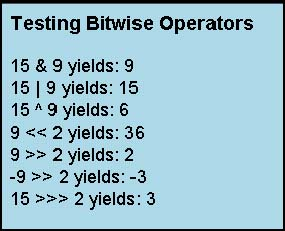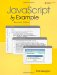JavaScript by Example (2nd Edition)
ISBN: 0137054890
EAN: 2147483647
Year: 2003
Pages: 150
Authors: Ellie Quigley

Similar book on Amazon